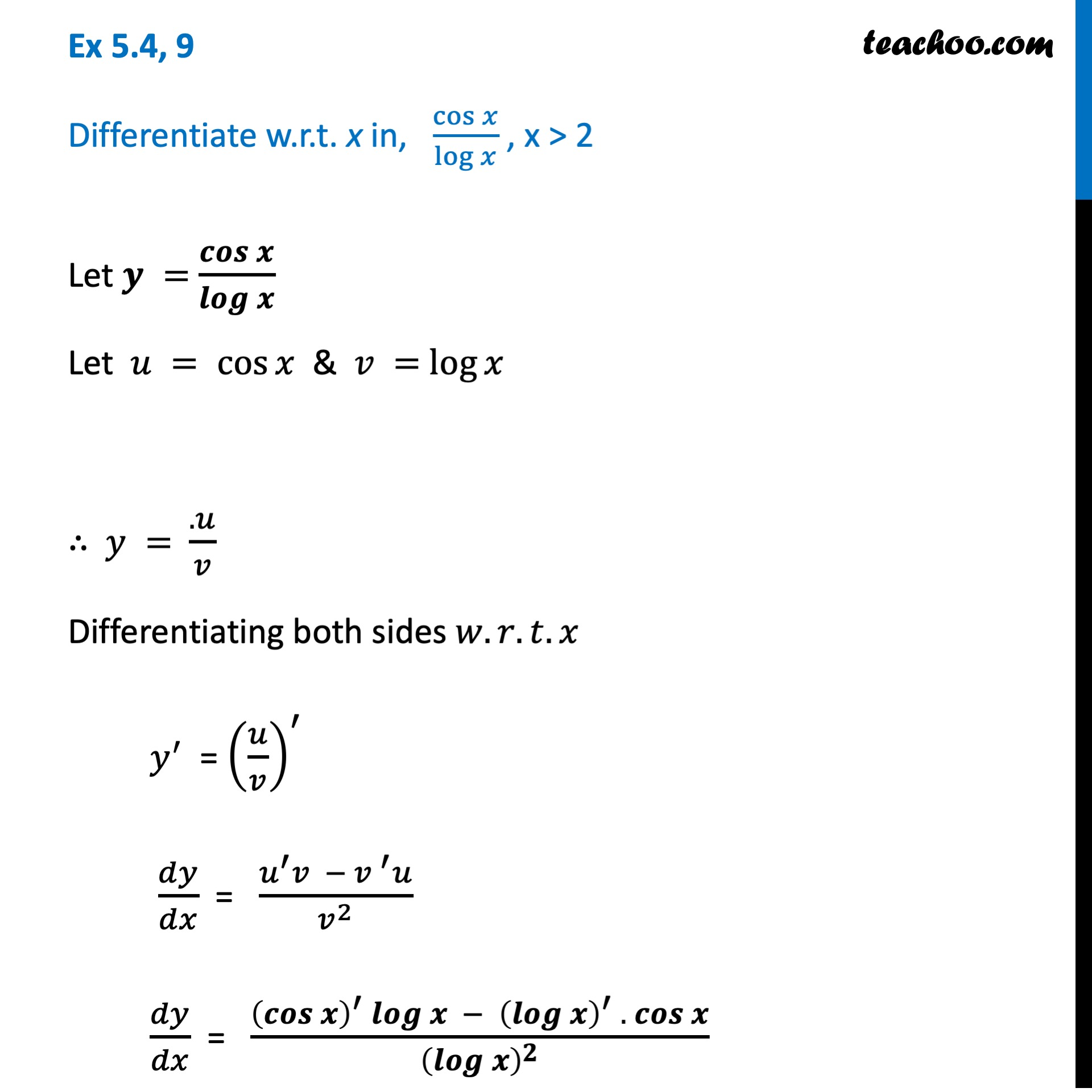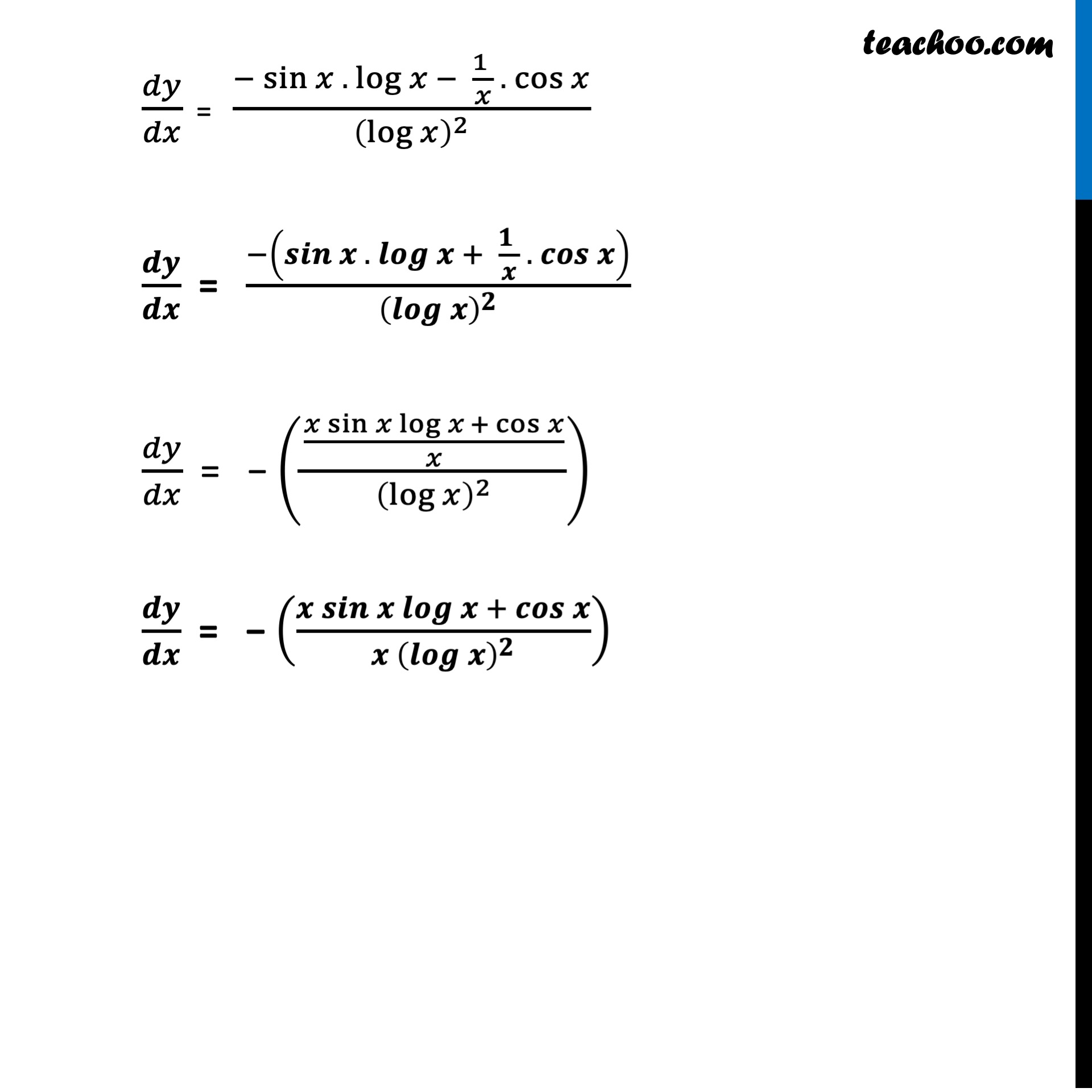Ex 5.4

Chapter 5 Class 12 Continuity and Differentiability
Serial order wiseLearn in your speed, with individual attention - Teachoo Maths 1-on-1 Class

### Transcript

Ex 5.4, 9 Differentiate w.r.t. x in, cos⁡𝑥/log⁡𝑥 , x > 2Let 𝑦 = cos⁡𝑥/log⁡𝑥 Let 𝑢 = cos⁡𝑥 & 𝑣 = log⁡𝑥 ∴ 𝑦 = (.𝑢)/𝑣 Differentiating both sides 𝑤.𝑟.𝑡.𝑥 𝑦^′ = (𝑢/𝑣)^′ 𝑑𝑦/𝑑𝑥 = (𝑢^′ 𝑣 − 〖𝑣 〗^′ 𝑢)/𝑣^2 𝑑𝑦/𝑑𝑥 = ((cos⁡𝑥 )^′ log⁡𝑥 − (log⁡𝑥 )^′ . 〖 cos〗⁡𝑥)/(log⁡𝑥 )^2 𝑑𝑦/𝑑𝑥 = (−sin⁡𝑥 . log⁡𝑥 − (1 )/𝑥 . 〖 cos〗⁡𝑥)/(log⁡𝑥 )^2 𝑑𝑦/𝑑𝑥 = (−(sin⁡𝑥 . log⁡𝑥 + (1 )/𝑥 . 〖 cos〗⁡𝑥 ))/(log⁡𝑥 )^2 𝑑𝑦/𝑑𝑥 = − (((𝑥 sin⁡〖𝑥 log⁡〖𝑥 + cos⁡𝑥 〗 〗)/𝑥)/(log⁡𝑥 )^2 ) 𝒅𝒚/𝒅𝒙 = − ((𝒙 𝒔𝒊𝒏⁡〖𝒙 𝒍𝒐𝒈⁡〖𝒙 +〖 𝒄𝒐𝒔〗⁡𝒙 〗 〗)/(𝒙 (𝒍𝒐𝒈⁡𝒙 )^𝟐 ))